My name is holly i am a tenth grader at bonita vista high school in san diego california. This is a secondary question. I am a student. What is the formoula for finding the arc length of a central angle of a circle?? Hi Holly, If you consider a circle with radius r then its circumference is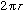. Thus when the angle is 360o the arclength is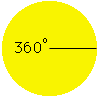Arclength =If the angle is less than 360o, that is some fraction of 360o, then the arclength is the same fraction of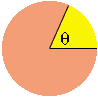Arclength =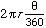If you measure the angle in radians rather than degrees then the expression is simpler. Since 360o is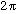radians, the arclength in the second diagram is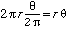. Cheers,Harley Go to Math Central